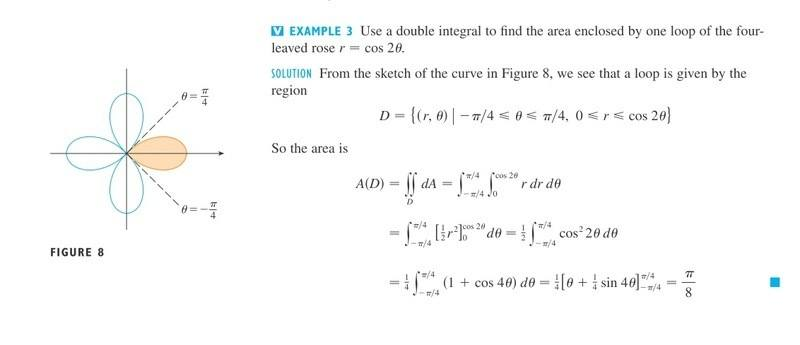# Double integral for loop of rose r=cos2θ

## Homework Statement## The Attempt at a Solution

Solution is given.

I don't understand how +-∏/4 is found as a range for θ
Also why is 0 <= r <= cos2θ

r is always r which is defined as cos2θ

LCKurtz
Homework Helper
Gold Member

## The Attempt at a Solution

Solution is given.
Where? I don't see it.

I don't understand how +-∏/4 is found as a range for θ
Did you draw the graph or have one to look at?

Also why is 0 <= r <= cos2θ

r is always r which is defined as cos2θ

That is r on the curve. If you want the area enclosed, r goes from r=0 to r on the curve, no?

Last edited by a moderator:
LCKurtz